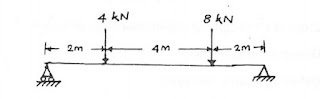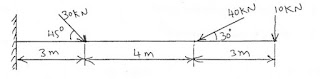# Strength of Materials Diploma M Scheme Important questions Unit – 1

Strength of Materials Diploma M Scheme Important questions

Dear Faculty / Students

We added Strength of Materials Unit 1 important questions

study well, best wishes.

Thank you

Subject : Strength of Materials

UNIT – 1, Statics of particle and Friction              Scheme : M

NOTE : 1. The highlighted Questions are asked more than two times in the Board Exam

1. These Questions are collected from Board Examination Question Papers.

2/3 marks Questions

1. State the principle of transmissibility of forces.
2. Define limiting forces
3. State parallelogram law of forces.
4. State Varignon’s theorem.
5. Write down the conditions of equilibrium.
6. What is dynamic friction?
7. State triangular law of forces
8. Distinguish between moment and couple.
9. Define angle of friction.
10. Explain internal forces & external forces
11. Explain cone of friction.
12. Define resultant of forces.
13. What is meant by coplanar force?
14. Differentiate between static friction & dynamic friction.
15. What are the characteristics of force?
16. Distinguish between force of friction and limiting force of friction.
17. Define co-efficient of friction.10 marks questions

1. Five forces acting on a particle. The magnitude of the forces are 300N, 600N, 700N, 900N and ‘P’ and their respective angles with the horizontal are 0 degree, 60 degree, 135 degree, 20 degree, and 270 degree. If the vertical component of all the forces is -1000N, find the value of ‘P’. Also calculate the magnitude and the direction of the resultant force assuming that 300N force act towards the particle where all others act away from the particle.
2. Determine the support reactions of the beam shown in the figure.1.  A particle ‘O’ is acted upon the following forces.
2.     20N inclined 30 degree to north of east.  25N towards the north.

iii.    30N towards north-west., 35N inclined 40 degree to south of west.

Find the magnitude and direction of the resultant force.

1. Discuss the various types of supports with neat sketches and show the reaction components of each.
2. The magnitude of the resultant of two concurrent forces including an angle of 90 degree between them is square root of 13 kN. When the included angle between the forces is 60 degree, the magnitude of their resultant is square root of 19 kN. Find the magnitude of the two forces.
3. Explain the various methods of supports and reactions.
4. State the laws of dynamic friction.
5. The resultant of two concurrent forces is 1500N and angle between the forces is 90 degree. The resultant makes an angle of 36 degree with the force. Find the magnitude of each force.
6. What are the essential conditions for equilibrium of rigid body?
7. State the laws of static friction.
8. The magnitude of the resultant of two concurrent forces including an angle of 90 degree between them is square root of 3 kN. When the included angle between the forces is 60 degree, the magnitude of their resultant is square root of 19 kN. Find the magnitude of the two forces.
9. Find the support reactions and moment at fixed end of a cantilever beam as shown in figure.